# Probability Practice Set For RBI Grade B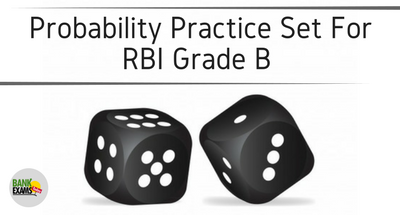## Question Set-1

Bankexamtoday wants to open his office in India. There are 40% & 60% chance that their office will be in Haryana & Punjab respectively. If they open the office in Haryana, there is 40% chance that it will be in Panchkula & 60% chance that it will be in Chandigarh. If they start an office in Punjab, there are 50%, 25% & 25% chances that it will be in Ludhiana, Amritsar & Chandigarh respectively.

### Ques.1:

Find the probability of opening the office in Ludhiana?
(a) 1/5
(b) 3/10
(c) 4/10
(d) 6/10
(e) none of these.

### Ques.2:

What is the probability of opening the office in Chandigarh from any of those two states?
(a) 39/100
(b) 2/10
(c) 2/5
(d) 4/9
(e) none of these.

## Question Set-2:

There are two sets of letters & we have to choose exactly one letter from each set.
Set A: {A, B, C, D, E}
Set B: {U, V, W, X, Y, Z}

### Ques.1:

What is the probability of choosing a D and X?
(a) 1/30
(b) 2/30
(c) 3/30
(d) 4/30
(e) none of these.

### Ques.2:

What is the probability of choosing a D or X?
(a) 1/2
(b) 1/3
(c) ¼
(d) 1/5
(e) none of these.

### Ques.3:

What is the probability of choosing two vowels?
(a) 1/15
(b) 2/15
(c) 3/15
(d) 4/15
(e) none of these

### Ques.4:

What is the probability of choosing at least one vowel?
(a) 3/2
(b) 1
(c) 1/2
(d) 1/3
(e) none of these.

## Question Set3.

Tiger has 4 books of Arithmetic, 3 books of advanced-math & 2 books of combine metrics. Out of these books, he selects two books at random one after other. What is the probability that he selects one Arithmetic & one combine metrics book?
(a) 1/9
(b) 2/9
(c) 3/9
(d) 4/9
(e) none of these.

## Question Set4:

Raj has two bags viz. Bag A & Bag B. Each bag contains balls of two colours viz. red and green. Given that, Bag A contains 3 red balls & 4 green balls and bag B contains 4 red balls & 5 green balls.

### Ques.1.

Raj has to select one bag randomly and pick a ball from that. What is the probability that ball drawn is green in colour?
(a) 67/126
(b) 69/126
(c) 71/126
(d) 73/126
(e) none of these.

### Ques.2.

Simran, a friend of raj transferred a ball from bag A to bag B, then raj draws a ball from bag B. What is the probability that ball drawn is green in colour?
(a) 69/126
(b) 38/75
(c) 35/69
(d) 39/70
(e) none of these.

### Ques.3.

Simran, a friend of raj transferred a ball from bag A to bag B, then raj draws a ball from bag B. Find the probability that the transferred ball is green, given that the ball drawn is green in colour?
(a) 5/17
(b) 8/13
(c) 7/13
(d) 5/24
(e) none of these.

### Ans. 1.

The probability of opening the office in Ludhiana= P (office in Punjab) ×P (office in Ludhiana)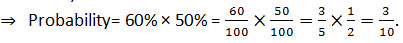### Ans. 2.

The probability of opening the office in Chandigarh
= P( Office in Chandigarh from Haryana) 0r P (Office in Chandigarh from Punjab)### Ans. 1.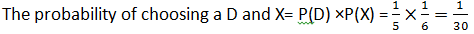### Ans. 2.

The probability of choosing a D or X = P(D or X) = P(D)+P(X)-P(D∩X)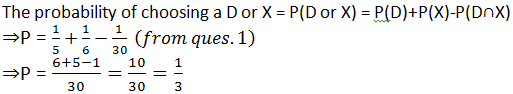### Ans. 3.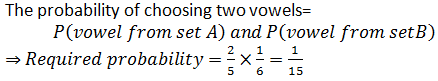### Ans. 4.

The probability of choosing at least one vowel= 1- probability of choosing no vowel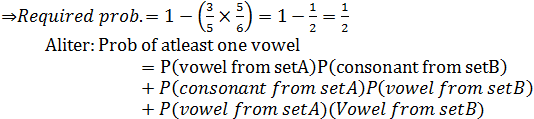The probability of selecting one Arithmetic & one combine metrics book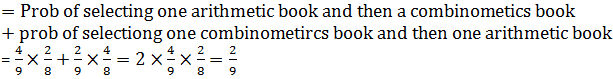## Question Set4:

### Ans. 1.

Required probability = P(selecting bag A & drawing green ball) or
P(selecting bag B & drawing a green ball) =### Ans. 2.

If a red ball is transferred, then bag B will have 5 red balls & 5 green balls.
Probability that green ball is drawn=#### What's trending in BankExamsToday

Smart Prep Kit for Banking Exams by Ramandeep Singh - Download here